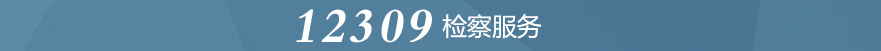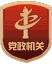1月24日，名山区检察院党组以“大力弘扬伟大建党精神，坚持和发展党的百年奋斗历史经验，坚定历史自信，践行时代使命，厚植为民情怀，勇于担当作为，团结带领人民群众走好新的赶考之路，为加快建设川藏铁路第一城、绿色发展示范市，全面建设社会主义现代化名山而努力奋斗”为主题，召开党史学习教育专题民主生活会。区委政法委副书记万萍、区委第五巡回指导组组长、区委组织部副部长赵丽华、市检察院三级高级警长李茂强等到会指导，名山区检察院党组书记、检察长郝连忠主持会议。
• []
• []
• []
• []
• []
• []
• []

• []
• []
• []
• []
• []
• []
• []

• []
• []
• []
• []
• []
• []
• []

• []
• []
• []
• []
• []
• []
• []• []
• []
• []
• []
• []
• []
• []

• []
• []
• []
• []
• []
• []
• []